Updating search results...

# 28 Results

View
Selected filters:
• MCCRS.Math.Content.8.EE.C.7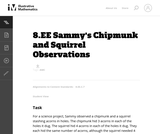Unrestricted Use
CC BY
Rating
0.0 stars

This is a task from the Illustrative Mathematics website that is one part of a complete illustration of the standard to which it is aligned. Each task has at least one solution and some commentary that addresses important asects of the task and its potential use. Here are the first few lines of the commentary for this task: For a science project, Sammy observed a chipmunk and a squirrel stashing acorns in holes. The chipmunk hid 3 acorns in each of the holes it dug. The sq...

Subject:
Mathematics
Material Type:
Activity/Lab
Provider:
Illustrative Mathematics
Provider Set:
Illustrative Mathematics
Author:
Illustrative Mathematics
Date Added:
05/19/2013Conditional Remix & Share Permitted
CC BY-NC
Rating
0.0 stars

students will work on knowledge check

Subject:
Mathematics
Material Type:
Lesson Plan
Author:
Harrison Wicker
Date Added:
08/14/2017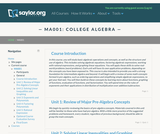Unrestricted Use
CC BY
Rating
4.0 stars

This course is also intended to provide the student with a strong foundation for intermediate algebra and beyond. Upon successful completion of this course, you will be able to: simplify and solve linear equations and expressions including problems with absolute values and applications; solve linear inequalities; find equations of lines; and solve application problems; add, subtract, multiply, and divide various types of polynomials; factor polynomials, and simplify square roots; evaluate, simplify, multiply, divide, add, and subtract rational expressions, and solve basic applications of rational expressions. This free course may be completed online at any time. It has been developed through a partnership with the Washington State Board for Community and Technical Colleges; the Saylor Foundation has modified some WSBCTC materials. (Mathematics 001)

Subject:
Algebra
Material Type:
Full Course
Provider:
The Saylor Foundation
Date Added:
04/16/2012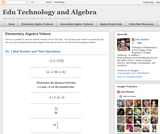Conditional Remix & Share Permitted
CC BY-NC
Rating
0.0 stars

Visually searchable database of Algebra 1 videos. Click on a problem and watch the solution on YouTube. Copy and paste this material into your CMS. Videos accompany the open Elementary Algebra textbook published by Flat World Knowledge.

Subject:
Algebra
Material Type:
Lecture
Lecture Notes
Provider:
Individual Authors
Provider Set:
Individual Authors
Author:
John Redden
Date Added:
04/29/2012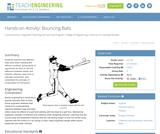Read the Fine Print
Educational Use
Rating
0.0 stars

Students examine how different balls react when colliding with different surfaces, giving plenty of opportunity for them to see the difference between elastic and inelastic collisions, learn how to calculate momentum, and understand the principle of conservation of momentum.

Subject:
Engineering
Physics
Material Type:
Activity/Lab
Provider:
TeachEngineering
Provider Set:
TeachEngineering
Author:
Bailey Jones
Chris Yakacki
Denise Carlson
Malinda Schaefer Zarske
Matt Lundberg
Date Added:
10/14/2015Only Sharing Permitted
CC BY-NC-ND
Rating
3.0 stars

This lesson unit is intended to help teachers assess how well students are able to create and solve linear equations. In particular, the lesson will help you identify and help students who have the following difficulties: solving equations with one variable and solving linear equations in more than one way.

Subject:
Algebra
Material Type:
Assessment
Lesson Plan
Provider:
Shell Center for Mathematical Education
Provider Set:
Mathematics Assessment Project (MAP)
Date Added:
04/26/2013Only Sharing Permitted
CC BY-NC-ND
Rating
4.0 stars

This lesson unit is intended to help teachers assess how well students are able to classify solutions to a pair of linear equations by considering their graphical representations. In particular, this unit aims to help teachers identify and assist students who have difficulties in: using substitution to complete a table of values for a linear equation; identifying a linear equation from a given table of values; and graphing and solving linear equations.

Subject:
Algebra
Material Type:
Assessment
Lesson Plan
Provider:
Shell Center for Mathematical Education
Provider Set:
Mathematics Assessment Project (MAP)
Date Added:
04/26/2013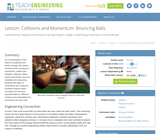Read the Fine Print
Educational Use
Rating
0.0 stars

As a continuation of the theme of potential and kinetic energy, this lesson introduces the concepts of momentum, elastic and inelastic collisions. Many sports and games, such as baseball and ping-pong, illustrate the ideas of momentum and collisions. Students explore these concepts by bouncing assorted balls on different surfaces and calculating the momentum for each ball.

Subject:
Engineering
Physics
Material Type:
Activity/Lab
Lesson Plan
Provider:
TeachEngineering
Provider Set:
TeachEngineering
Author:
Bailey Jones
Chris Yakacki
Denise Carlson
Malinda Schaefer Zarske
Matt Lundberg
Date Added:
09/18/2014Unrestricted Use
CC BY
Rating
0.0 stars

This task involves solving equations with rational coefficients, and requires students to use the distributive law ("combine like terms"). The equation also provides opportunities for students to observe structure in the equation to find a quicker solution, as in the second solution presented.

Subject:
Mathematics
Material Type:
Activity/Lab
Provider:
Illustrative Mathematics
Provider Set:
Illustrative Mathematics
Author:
Illustrative Mathematics
Date Added:
05/01/2012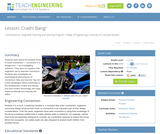Read the Fine Print
Educational Use
Rating
0.0 stars

Students learn about the physical force of linear momentum movement in a straight line by investigating collisions. They learn an equation that engineers use to describe momentum. Students also investigate the psychological phenomenon of momentum; they see how the "big mo" of the bandwagon effect contributes to the development of fads and manias, and how modern technology and mass media accelerate and intensify the effect.

Subject:
Engineering
Physics
Material Type:
Activity/Lab
Lesson Plan
Provider:
TeachEngineering
Provider Set:
TeachEngineering
Author:
Ben Heavner
Chris Yakacki
Denise Carlson
Malinda Schaefer Zarske
Date Added:
09/18/2014Unrestricted Use
CC BY
Rating
0.0 stars

Explore what it means for a mathematical statement to be balanced or unbalanced by interacting with objects on a balance. Discover the rules for keeping it balanced. Collect stars by playing the game!

Subject:
Mathematics
Material Type:
Simulation
Provider:
University of Colorado Boulder
Provider Set:
PhET Interactive Simulations
Author:
David Wood
Marleene Buttice
Meenakshi Raghavan
Date Added:
03/06/2019Only Sharing Permitted
CC BY-NC-ND
Rating
4.0 stars

This lesson unit is intended to help teachers assess how well students are able to: estimate lengths of everyday objects; convert between decimal and scientific notation; and make comparisons of the size of numbers expressed in both decimal and scientific notation.

Subject:
Mathematics
Material Type:
Assessment
Lesson Plan
Provider:
Shell Center for Mathematical Education
Provider Set:
Mathematics Assessment Project (MAP)
Date Added:
04/26/2013Only Sharing Permitted
CC BY-NC-ND
Rating
3.0 stars

This lesson unit is intended to help you assess how well students working with square numbers are able to: choose an appropriate, systematic way to collect and organize data, examining the data for patterns; describe and explain findings clearly and effectively; generalize using numerical, geometrical, graphical and/or algebraic structure; and explain why certain results are possible/impossible, moving towards a proof.

Subject:
Algebra
Geometry
Measurement and Data
Material Type:
Assessment
Lesson Plan
Provider:
Shell Center for Mathematical Education
Provider Set:
Mathematics Assessment Project (MAP)
Date Added:
04/26/2013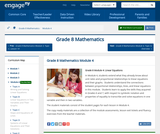Conditional Remix & Share Permitted
CC BY-NC-SA
Rating
0.0 stars

In Module 4, students extend what they already know about unit rates and proportional relationships to linear equations and their graphs.  Students understand the connections between proportional relationships, lines, and linear equations in this module.  Students learn to apply the skills they acquired in Grades 6 and 7, with respect to symbolic notation and properties of equality to transcribe and solve equations in one variable and then in two variables.

Subject:
Algebra
Material Type:
Module
Provider:
New York State Education Department
Provider Set:
EngageNY
Date Added:
11/12/2013Unrestricted Use
CC BY
Rating
0.0 stars

My goal is to merge New York State standards with Common Core Standards and Integrated Algebra Regent Standards for our 8th grade curriculum.

Subject:
Mathematics
Material Type:
Full Course
Lesson Plan
Syllabus
Author:
Shaun Errichiello
Date Added:
01/28/2016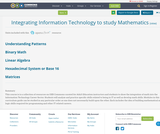Conditional Remix & Share Permitted
CC BY-SA
Rating
0.0 stars

This course is is a collection of resources on OER Commons curated for Adult Education instructors and students to show the integration of math into the Information Technology Career Sector. Students will analyze and practice specific skills related to being in IT as well as develop math skills. Modules in this curriculum guide can be studied in any particular order as one does not necessarily build upon the other. Each includes the idea of building mathematical and logic skills required for programming and other IT related careers.

Subject:
Career and Technical Education
Mathematics
Material Type:
Teaching/Learning Strategy
Unit of Study
Author:
Lyn Michaud Smith
Date Added:
05/30/2018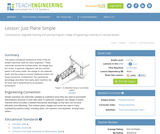Read the Fine Print
Educational Use
Rating
0.0 stars

This lesson introduces students to three of the six simple machines used by many engineers. These machines include the inclined plane, the wedge and the screw. In general, engineers use the inclined plane to lift heavy loads, the wedge to cut materials apart, and the screw to convert rotational motion into linear movement. Furthermore, the mechanical advantage describes how easily each machine can do work and is determined by its physical dimensions.

Subject:
Engineering
Physics
Material Type:
Activity/Lab
Lesson Plan
Provider:
TeachEngineering
Provider Set:
TeachEngineering
Author:
Jake Lewis
Janet Yowell
Malinda Schaefer Zarske
Date Added:
09/18/2014Only Sharing Permitted
CC BY-NC-ND
Rating
5.0 stars

This lesson unit is intended to help teahcers assess how well students are able to interpret speed as the slope of a linear graph and translate between the equation of a line and its graphical representation.

Subject:
Algebra
Material Type:
Assessment
Lesson Plan
Provider:
Shell Center for Mathematical Education
Provider Set:
Mathematics Assessment Project (MAP)
Date Added:
04/26/2013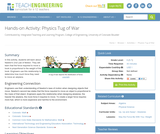Read the Fine Print
Educational Use
Rating
0.0 stars

In this activity, students will learn about Newton's 2nd Law of Motion. They will learn that the force required to move a book is proportional to the weight of the book. Engineers use this relationship to determine how much force they need to move an airplane.

Subject:
Engineering
Physics
Material Type:
Activity/Lab
Provider:
TeachEngineering
Provider Set:
TeachEngineering
Author:
Alex Conner
Geoffrey Hill
Janet Yowell
Malinda Schaefer Zarske
Tom Rutkowski
Date Added:
10/14/2015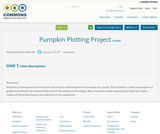Conditional Remix & Share Permitted
CC BY-NC
Rating
0.0 stars

Students create equations that have solutions to ordered pairs of an image on a graph. First students create an image on a graph and identify the ordered pairs for all the points of the image. Next, students create equations so that the x and y values of the ordered pairs are solutions to the equations.

Subject:
Education
Mathematics
Material Type:
Activity/Lab
Homework/Assignment
Author:
Rebecca Layton
Date Added:
07/25/2016